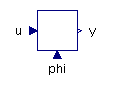Circular Pipe - MapleSim Help

Circular Pipe

Lossy model of a circular pipeDescription The Circular Pipe component models a circular pipe with losses. The pressure drop is computed with the Darcy equation, with the friction factor determined using the Haaland approximation for turbulent flow. When the Reynolds number is greater than the maximum value for laminar flow but less than the minimum value for turbulent flow, the friction factor is determined using linear interpolation.Equations $\mathrm{Re}=\frac{q\mathrm{D}}{a\mathrm{\nu }}\phantom{\rule[-0.0ex]{3.0ex}{0.0ex}}{\mathrm{D}}_{h}=4\frac{A}{U}\phantom{\rule[-0.0ex]{2.0ex}{0.0ex}}A=\frac{\mathrm{\pi }{\mathrm{D}}^{2}}{4}$ ${f}_{L}=\frac{64}{\mathrm{Re}}\phantom{\rule[-0.0ex]{2.5ex}{0.0ex}}{f}_{T}={f}_{\mathrm{Colebrook}}\left({\mathrm{Re}}_{T},\frac{\mathrm{\epsilon }}{{\mathrm{D}}_{h}}\right)$ $\mathrm{mode}=\left\{\begin{array}{cc}{\mathrm{pos}}_{\mathrm{turbulent}}& {\mathrm{Re}}_{T}<\mathrm{Re}\\ {\mathrm{neg}}_{\mathrm{turbulent}}& {\mathrm{Re}}_{T}<-\mathrm{Re}\\ {\mathrm{pos}}_{\mathrm{mixed}}\phantom{\rule[-0.0ex]{2.0ex}{0.0ex}}& {\mathrm{Re}}_{L}<\mathrm{Re}\\ {\mathrm{neg}}_{\mathrm{mixed}}\phantom{\rule[-0.0ex]{2.0ex}{0.0ex}}& {\mathrm{Re}}_{L}<-\mathrm{Re}\\ \mathrm{laminar}\phantom{\rule[-0.0ex]{3.5ex}{0.0ex}}& \mathrm{otherwise}\end{array}$ $p={p}_{A}-{p}_{B}=\frac{1}{2}L\mathrm{\rho }\frac{{\mathrm{\nu }}^{2}}{{\mathrm{D}}_{h}^{3}}\mathrm{Re}\left\{\begin{array}{cc}{f}_{\mathrm{Colebrook}}\left(\left|\mathrm{Re}\right|,\frac{\mathrm{\epsilon }}{{\mathrm{D}}_{h}}\right)\left|\mathrm{Re}\right|& \mathrm{mode}={\mathrm{pos}}_{\mathrm{turbulent}}\vee \mathrm{mode}={\mathrm{neg}}_{\mathrm{turbulent}}\\ \left({f}_{L}+\frac{{f}_{T}-{f}_{L}}{{\mathrm{Re}}_{T}-{\mathrm{Re}}_{L}}\left(\left|\mathrm{Re}\right|-{\mathrm{Re}}_{L}\right)\right)\left|\mathrm{Re}\right|& \mathrm{mode}={\mathrm{pos}}_{\mathrm{mixed}}\vee \mathrm{mode}={\mathrm{neg}}_{\mathrm{mixed}}\\ 64& \mathrm{otherwise}\end{array}$ $q={q}_{A}=-{q}_{B}=\mathrm{Re}A\frac{\mathrm{\nu }}{{\mathrm{D}}_{h}}$ ${f}_{\mathrm{Colebrook}}=\left(\mathrm{Re},{\mathrm{\epsilon }}_{\mathrm{D}}\right)\to {\left(1.8{\mathrm{log}}_{10}{\left(\frac{6.9}{\mathrm{Re}}+\left(\frac{{\mathrm{\epsilon }}_{\mathrm{D}}}{3.7}\right)\right)}^{1.11}\right)}^{-2}$Variables

 Name Units Description Modelica ID $A$ ${m}^{2}$ Pipe cross-sectional area A ${f}_{L}$ Friction factor with laminar flow fL ${f}_{T}$ Friction factor with turbulent flow fT $\mathrm{mode}$ Integer indicating type of flow mode $p$ $\mathrm{Pa}$ Pressure across component p $q$ $\frac{{m}^{3}}{s}$ Flow rate through component q $\mathrm{Re}$ Reynolds number ReConnections

 Name Description Modelica ID $\mathrm{portA}$ Upstream hydraulic port portA $\mathrm{portB}$ Downstream hydraulic port portBParametersGeneral Parameters

 Name Default Units Description Modelica ID $\mathrm{D}$ $0.01$ $m$ Inner diameter D $L$ $5$ $m$ Length of pipe L $\mathrm{\epsilon }$ $1.5·{10}^{-5}$ $m$ Height of inner surface roughness epsilon ${\mathrm{Re}}_{L}$ $2·{10}^{3}$ Reynolds number at transition to laminar flow ReL ${\mathrm{Re}}_{T}$ $4·{10}^{3}$ Reynolds number at transition to turbulent flow ReT